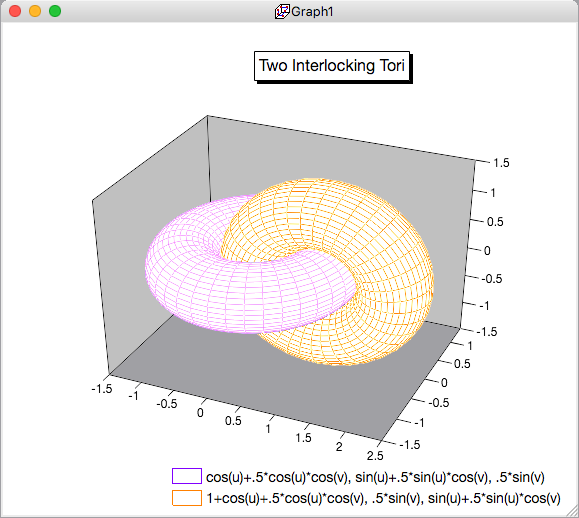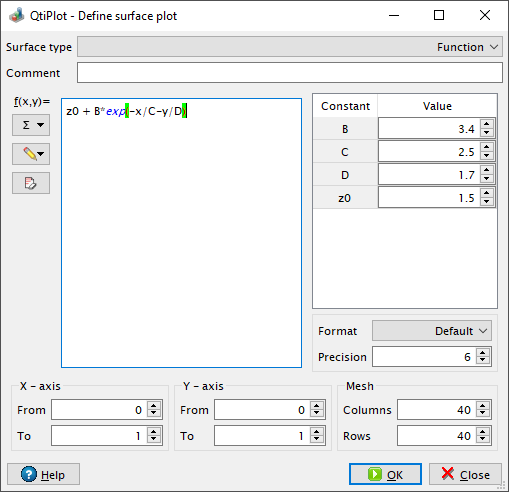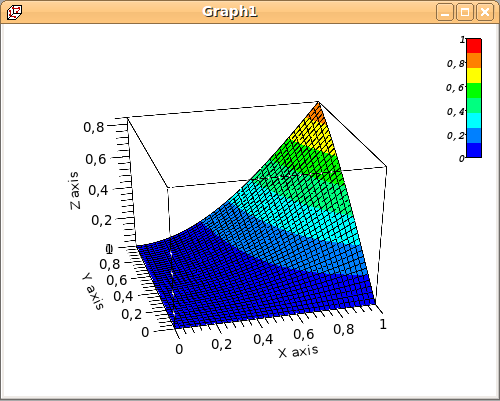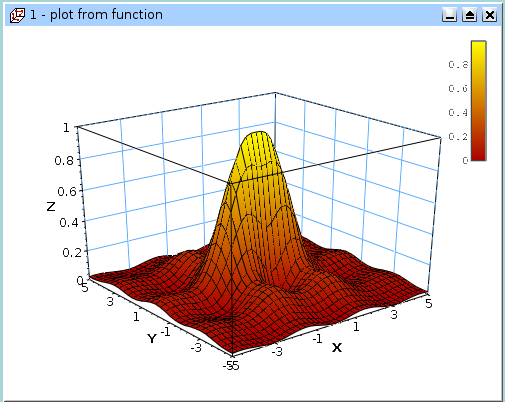# 3D plots

3D plots are generated from data defined as Z=f(X,Y). As with 2D plots, there are two ways to obtain a 3D plot, depending on the way the (X,Y,Z) values are defined:

• You can have your Z values in a matrix. QtiPlot will consider that all the data present in the matrix are Z values, and the X and Y values are defined as functions of the column and row numbers.

The data in the matrix can be entered in several ways:

• one by one from the keyboard,

• by reading an ASCII file into a table and converting the table into a matrix,

• by setting the values with a function.

• If you want to plot a function, you don't need a matrix. You can plot a function directly using the New 3D Surface Plot... command. This will open the corresponding dialog box where you define the mathematical expression of your function.

There are several kinds of 3D plots which can be selected, see the Plot 3D menu section of the reference chapter for a list of the available plots.

Figure 2-8. Example of a 3D Plots.3D plots use OpenGL so you can easily rotate, scale and shift them with the mouse. Using the 3D plot settings dialog or the Surface 3D Toolbar, you can change all the predefined settings of a three dimensional plot: grids, scales, axes, title, legend and colors for the different elements.

There are several types of plots which can be built from a matrix. They are presented in the Plot 3D menu

## Direct 3D plot from a function

This is the simplest way to obtain a 3d plot. Use the New 3D Surface Plot... command from the File menu or simply enter Ctrl-Alt-Z. This will open the following dialog box:

Figure 2-9. Definition of a new surface 3D plotYou can enter the function z=f(x,y) and the ranges for X, Y and Z. Then QtiPlot will create a default 3d plot:

Figure 2-10. The 3D surface plot created using defaultsYou can then customize the plot by opening the Surface plot options dialog. You can modify the axis ranges and parameters, add a title, change the colors of the different items, and modify the aspect ratio of the plot. In addition, you can use the commands of the 3D plot toolbar to add grids on the walls or to modify the style of the plot. The following plot illustrates some of the possible modifications:

Figure 2-11. The 3D surface plot after customization.If you want to modify the function itself, you can use the surface... command which can be activated from the context menu with a right click on the 3D plot. This will re-open the define surface function dialog box.

## 3D plot from a matrix

The second way to obtain a 3D plot is to use a matrix. Therefore, the first step is to fill the matrix. This can be done by evaluation of a function.

The New Matrix command create a default empty matrix with 32x32 cells. Then use the Set Dimensions... to modify the number of rows and columns of the matrix. This dialog box is also used to define the X and Y ranges.Then use the Set Values... command to fill the cells with numbers. The ranges of X and Y defined in the previous step are not known by this dialog box, so the function must be defined with row and column numbers (i and j) as parameters (see the section set-values for details).

The other way to obtain a matrix is to import an ASCII file into a table with the Import -> Import ASCII... command from the File menu. The table can then be transformed into a matrix with the Convert to Matrix from the Table menu.

You can then use this matrix to build a 3D plot with one of the commands from the Plot menu.